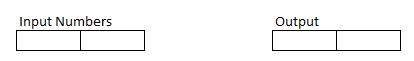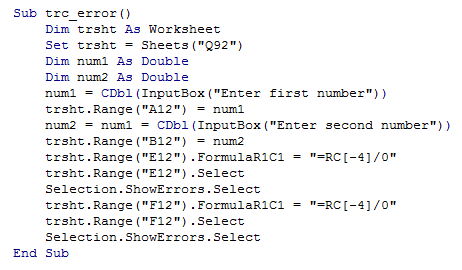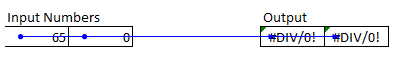## Vba trace error in vba in Excel

Trace Error in Excel lets you trace arrows back to cells referenced by a formula if it displays an error.�

The Trace Error�tool is available when auditing a worksheet within a workbook.

Note that the formula cell that you select must contain an error to use the Trace Error tool in Excel.

Invalid or inaccurate cell referencing is often a common cause of formula errors within Excel worksheets.

Using the Trace Error tool in Excel shows you the cells referenced by a selected formula that contains an error message.

If the cell referencing turns out to be the reason why the formula will not function, you can then fix the cell references within the formula cell to correct the error.

The following example demonstrates the Trace Error feature.The below code shows how to use the Trace Error technique in VBA.

For example purpose, the user has to input formula in such a way that will cause an error, so that, this feature can be demonstrated.

In our example, we have chosen a Divide by Zero Error, where in a number entered by the user in divided by zero, which will result in an error.

The numbers entered by the user are put in Input cells A12 and B12 and the corresponding output is shown in cells E12 and F12.

1. Sub trc_error()
2. Dim trsht As Worksheet
3. Set trsht = Sheets("Q92")
4. Dim num1 As Double
5. Dim num2 As Double
6. num1 = CDbl(InputBox("Enter first number"))
7. trsht.Range("A12") = num1
8. num2 = num1 = CDbl(InputBox("Enter second number"))
9. trsht.Range("B12") = num2
10. trsht.Range("E12").FormulaR1C1 = "=RC[-4]/0"
11. trsht.Range("E12").Select
12. Selection.ShowErrors.Select
13. trsht.Range("F12").FormulaR1C1 = "=RC[-4]/0"
14. trsht.Range("F12").Select
15. Selection.ShowErrors.Select
16. End Sub

The screenshots of editor and outputs are as shown below